# NCERT Solutions for Class 8 Maths Chapter 15 Exercise 15.1 – Introduction to Graphs

NCERT Solutions for Class 8 Maths Chapter 15 Exercise 15.1 – Introduction to Graphs, has been designed by the NCERT to test the knowledge of the student on the following topics:-

• A Bar graph
• A Pie graph (or a circle-graph)
• A histogram
• A line graph

### NCERT Solutions for Class 8 Maths Chapter 15 Exercise 15.1 – Introduction to Graphs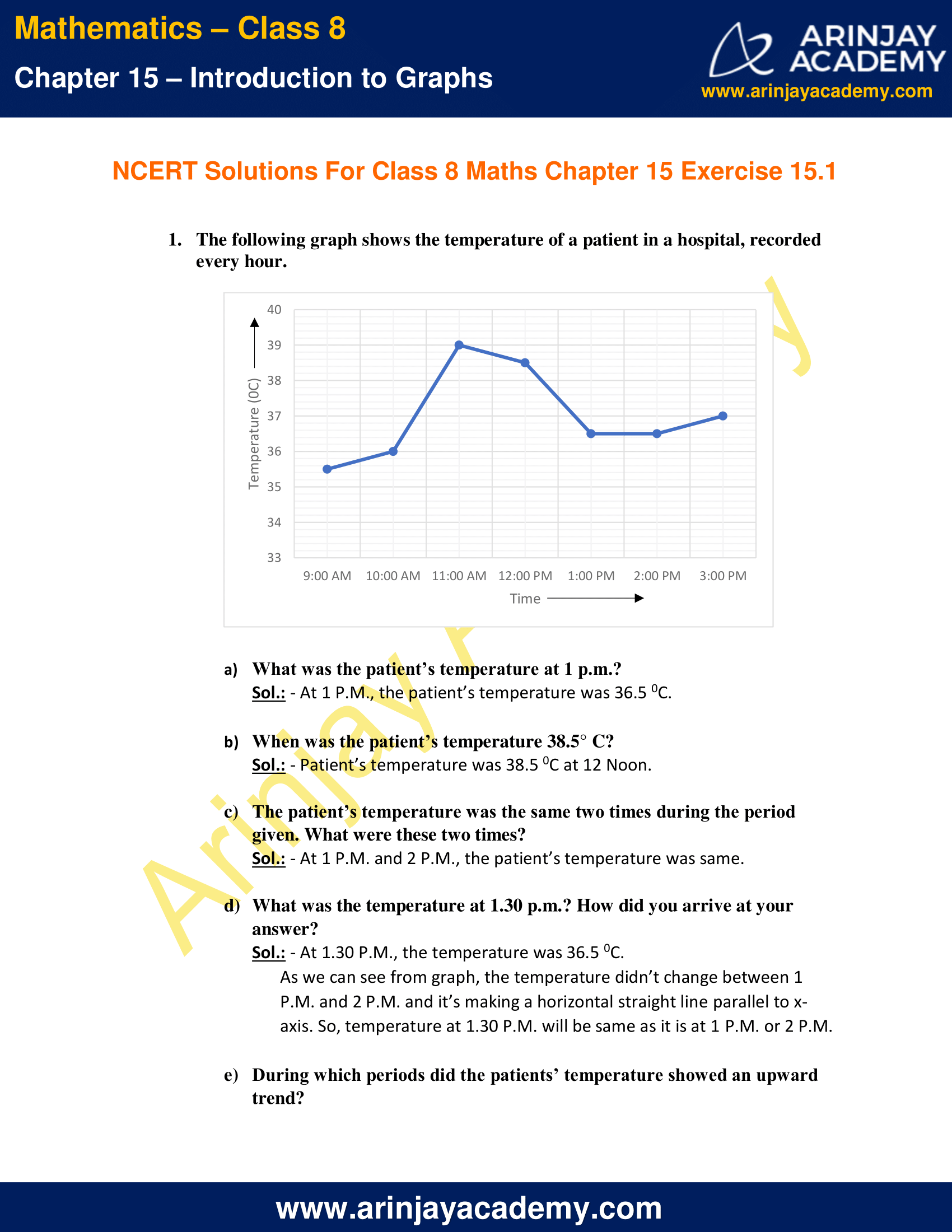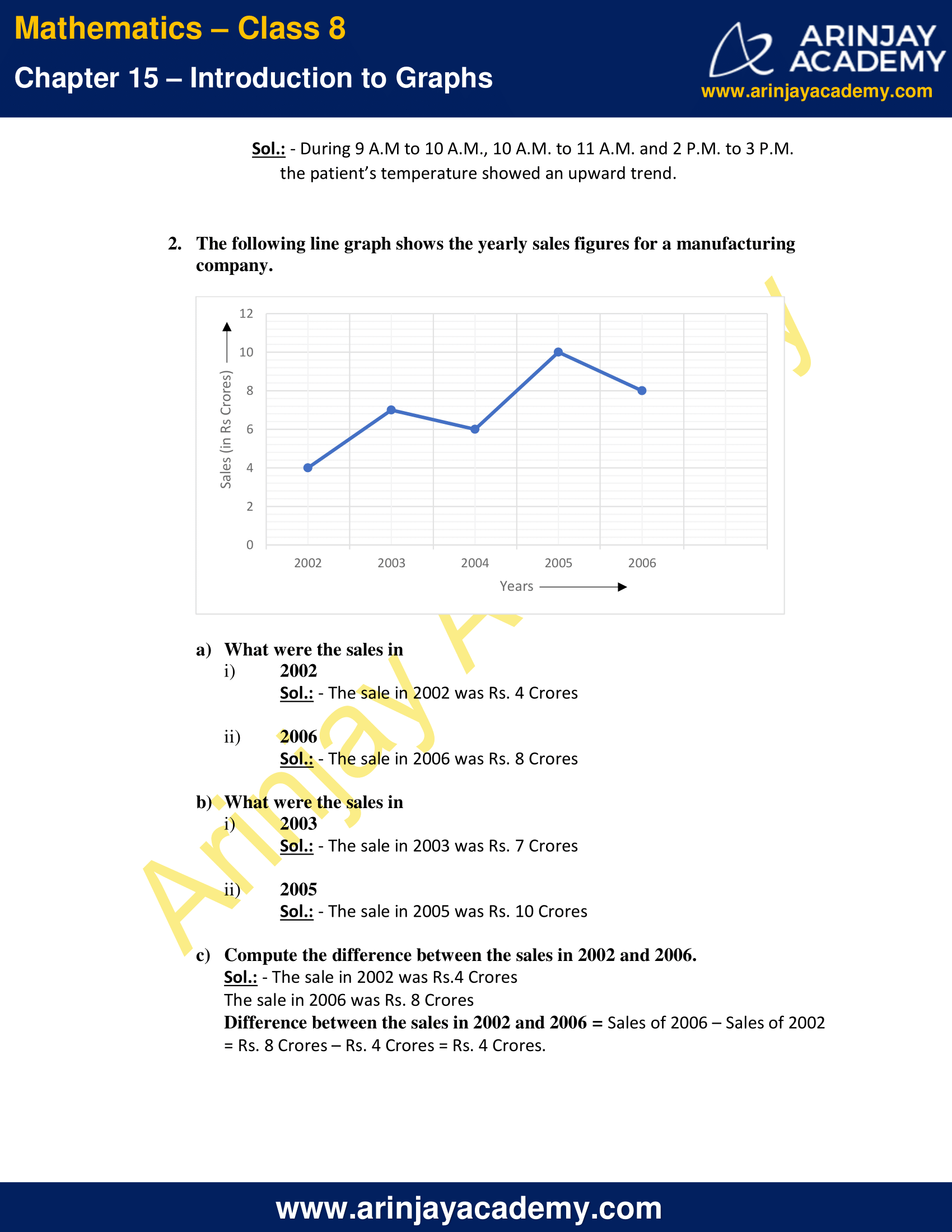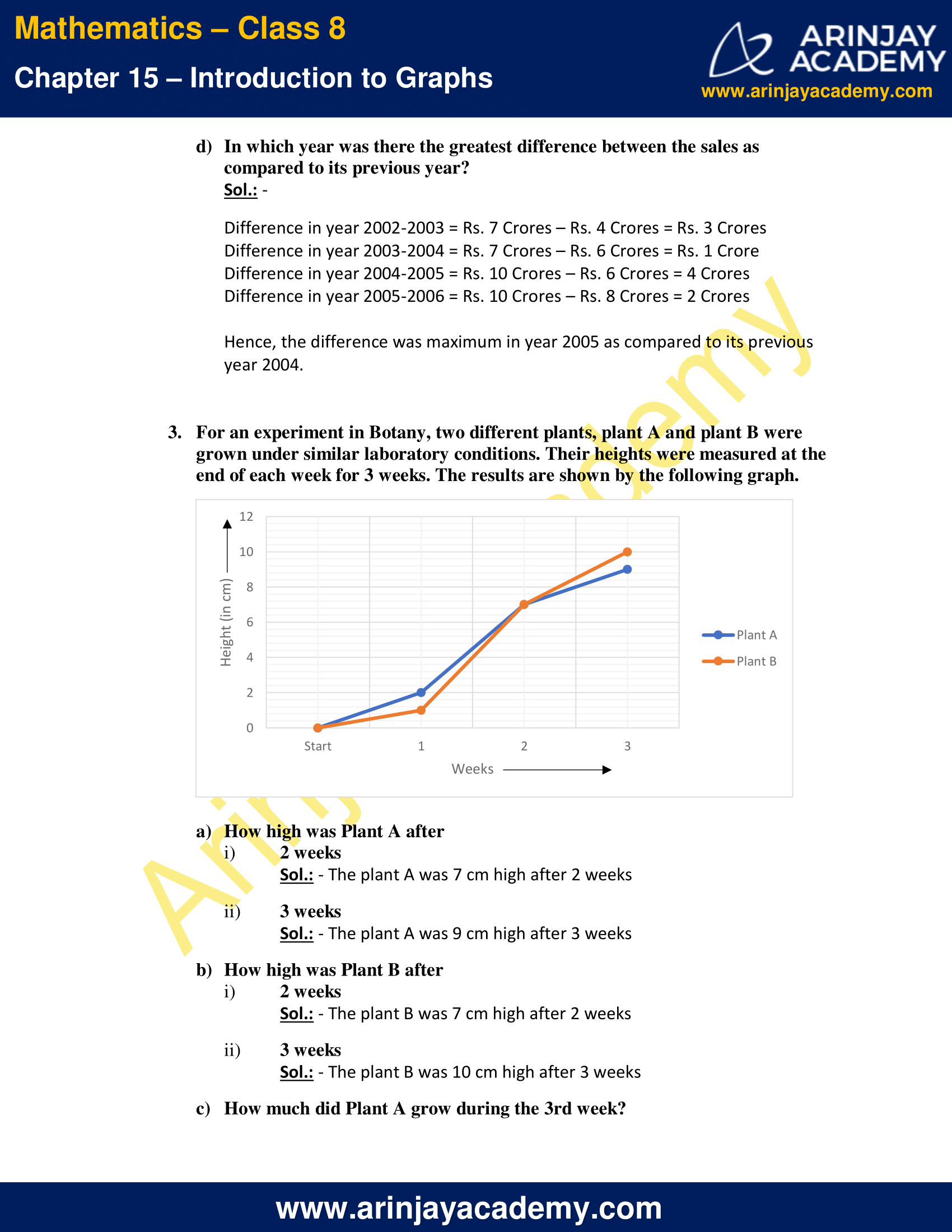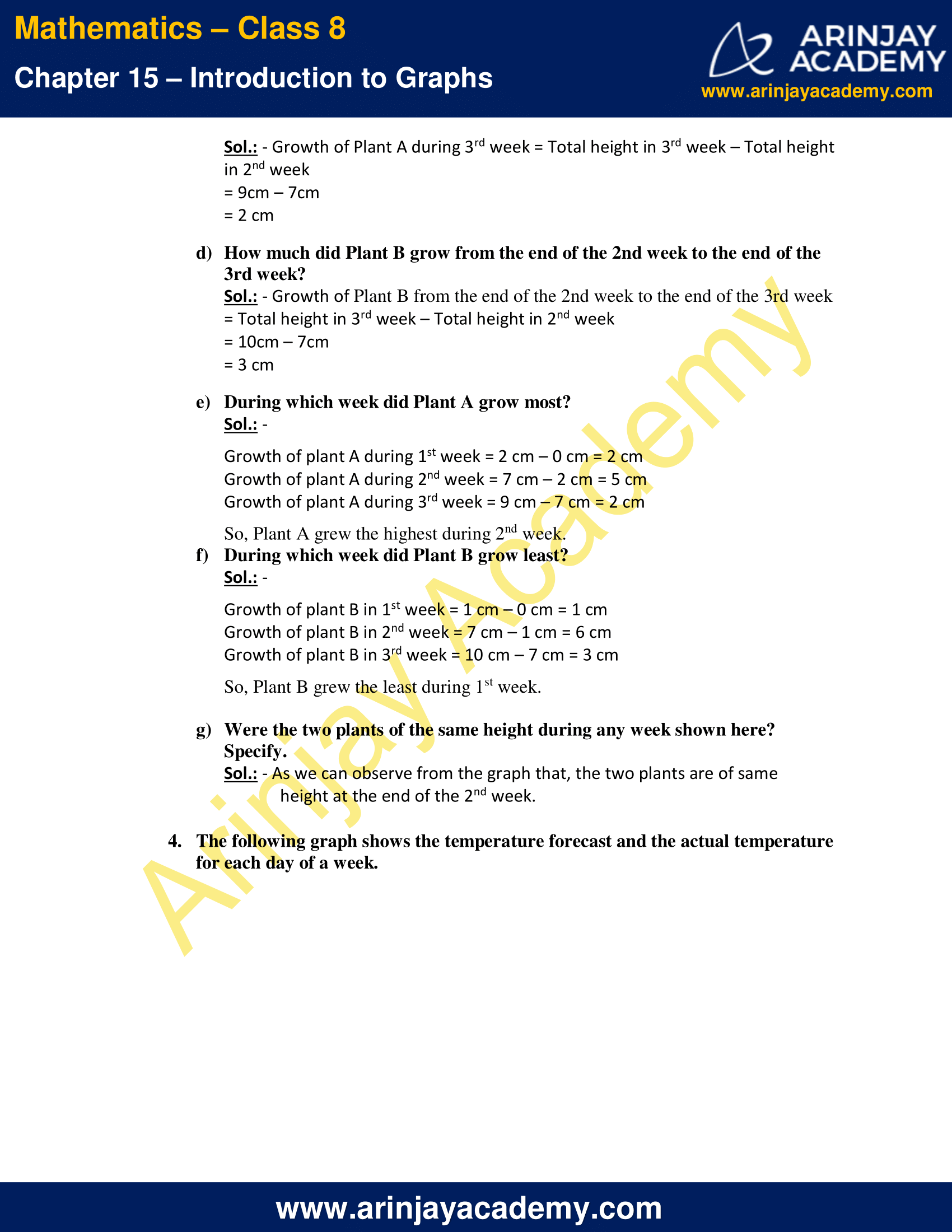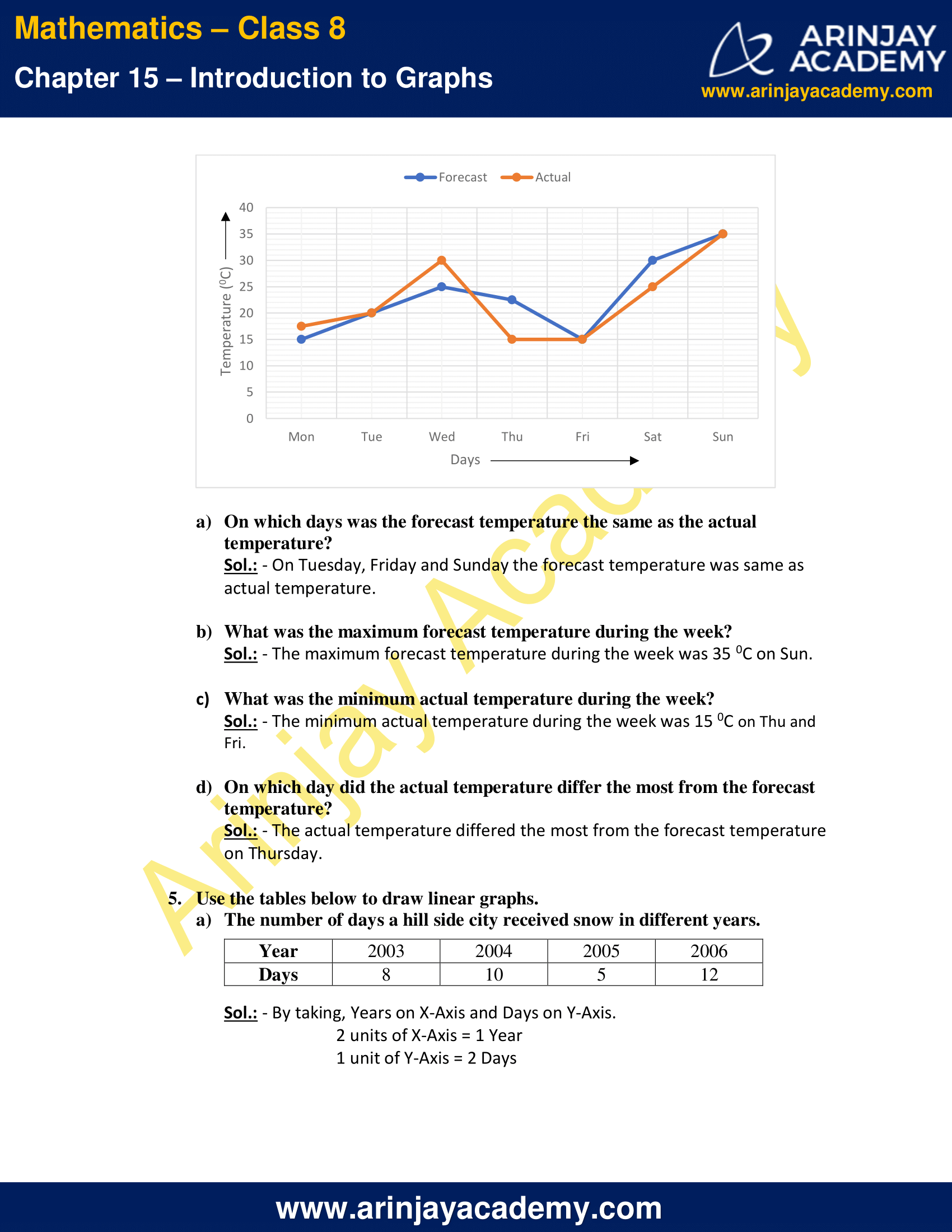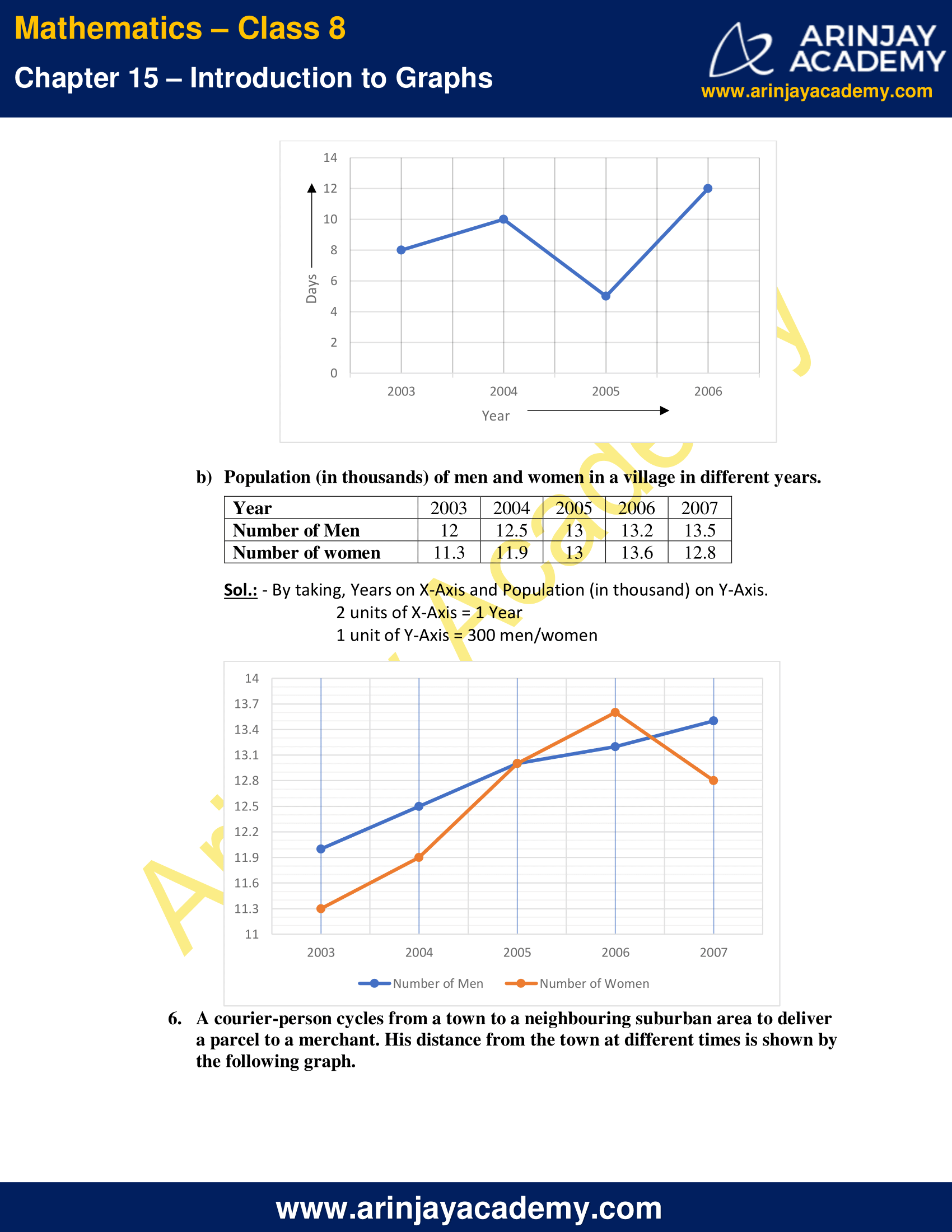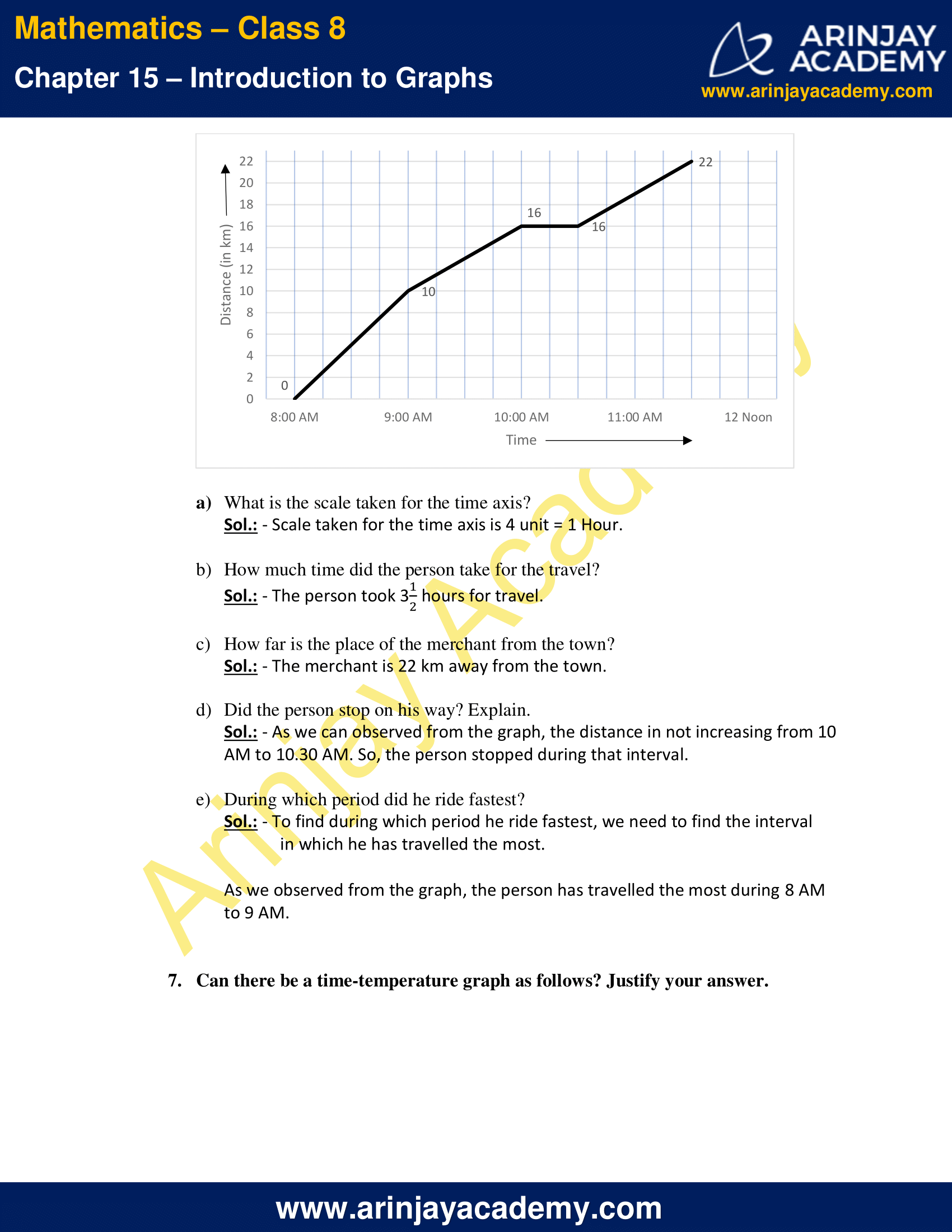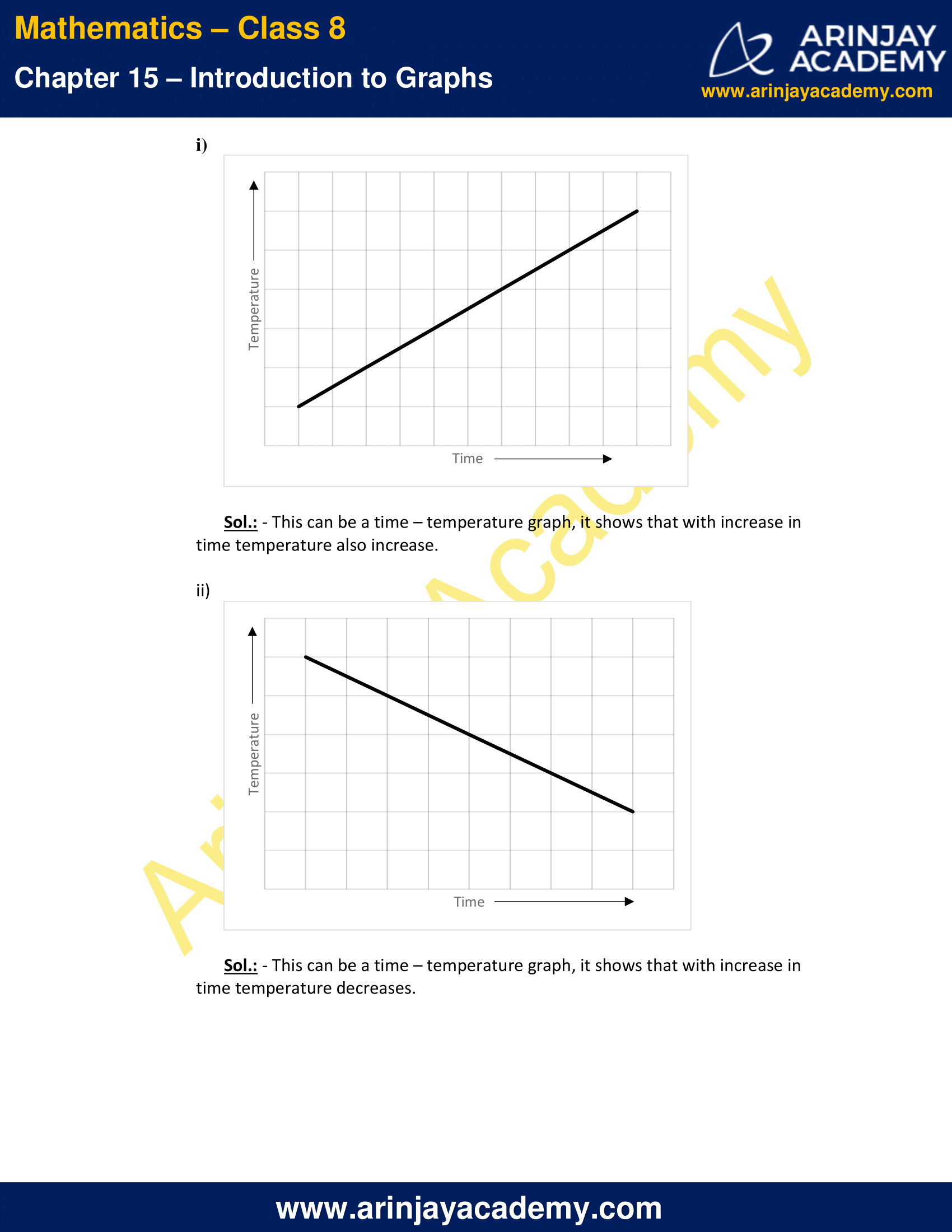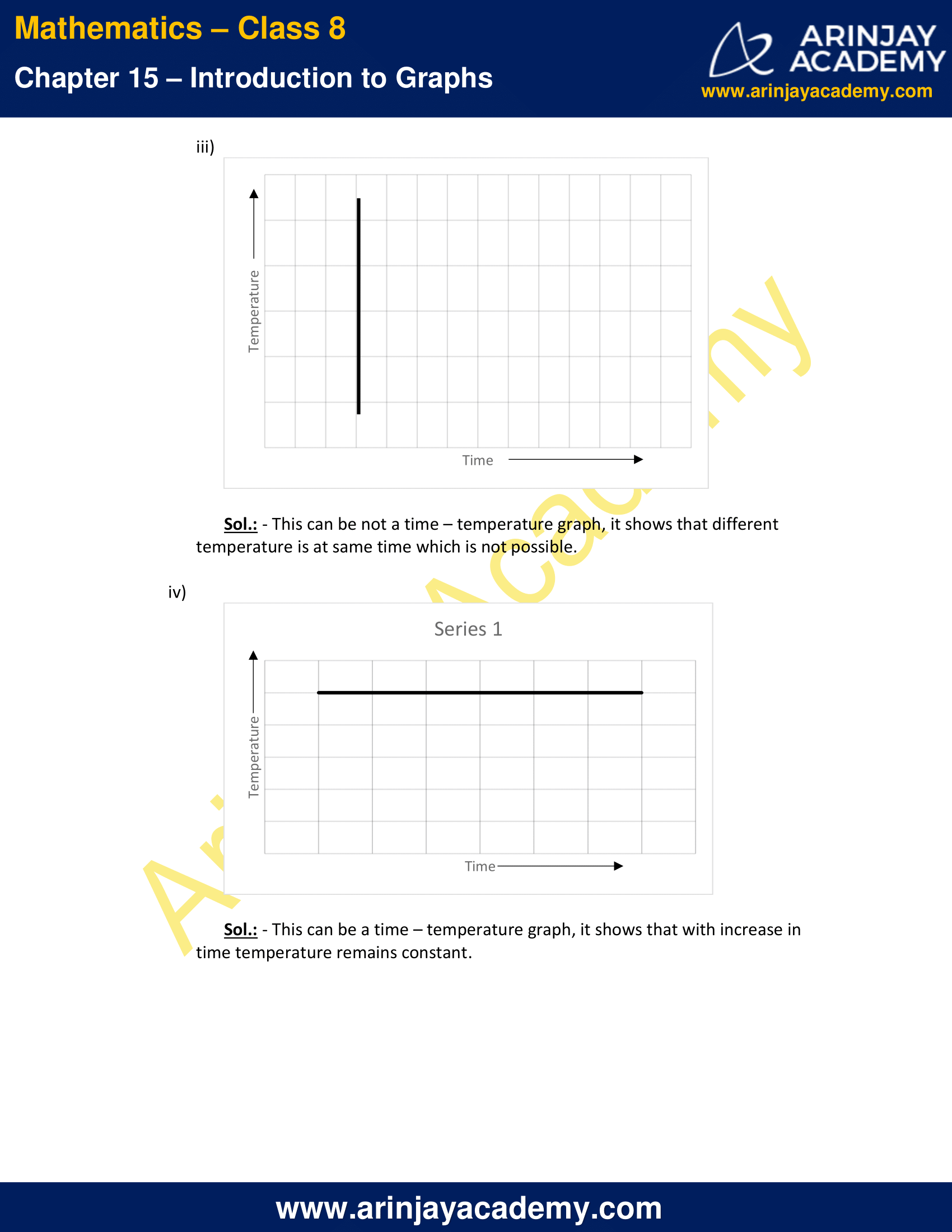NCERT Solutions for Class 8 Maths Chapter 15 Exercise 15.1 – Introduction to Graphs

1. The following graph shows the temperature of a patient in a hospital, recorded every hour.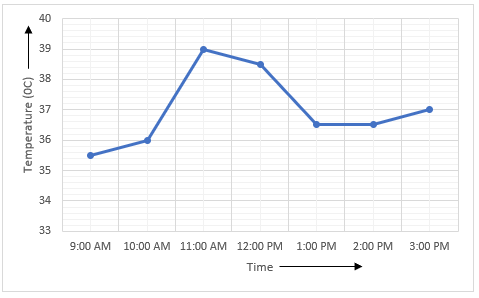a) What was the patient’s temperature at 1 p.m.?
Sol.: – At 1 P.M., the patient’s temperature was 36.5 0C.

b) When was the patient’s temperature 38.5° C?
Sol.: – Patient’s temperature was 38.5 0C at 12 Noon.

c) The patient’s temperature was the same two times during the period given. What were these two times?
Sol.: – At 1 P.M. and 2 P.M., the patient’s temperature was same.

d) What was the temperature at 1.30 p.m.? How did you arrive at your answer?
Sol.: – At 1.30 P.M., the temperature was 36.5 0C.
As we can see from graph, the temperature didn’t change between 1 P.M. and 2 P.M. and it’s making a horizontal straight line parallel to x-axis. So, temperature at 1.30 P.M. will be same as it is at 1 P.M. or 2 P.M.

e) During which periods did the patients’ temperature showed an upward trend?
Sol.: – During 9 A.M to 10 A.M., 10 A.M. to 11 A.M. and 2 P.M. to 3 P.M. the patient’s temperature showed an upward trend.

2. The following line graph shows the yearly sales figures for a manufacturing company.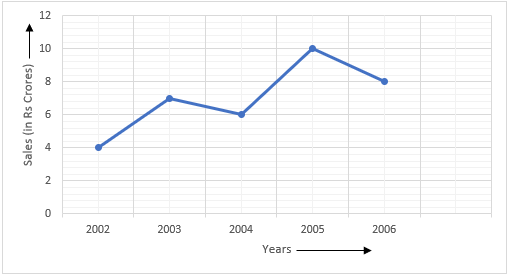a) What were the sales in

i) 2002
Sol.: – The sale in 2002 was Rs. 4 Crores

ii) 2006
Sol.: – The sale in 2006 was Rs. 8 Crores

b) What were the sales in

i) 2003
Sol.: – The sale in 2003 was Rs. 7 Crores

ii) 2005
Sol.: – The sale in 2005 was Rs. 10 Crores

c) Compute the difference between the sales in 2002 and 2006.

Sol.: – The sale in 2002 was Rs.4 Crores
The sale in 2006 was Rs. 8 Crores
Difference between the sales in 2002 and 2006
=
Sales of 2006 – Sales of 2002
= Rs. 8 Crores – Rs. 4 Crores = Rs. 4 Crores.

d) In which year was there the greatest difference between the sales as compared to its previous year?

Sol.:

Difference in year 2002-2003 = Rs. 7 Crores – Rs. 4 Crores = Rs. 3 Crores
Difference in year 2003-2004 = Rs. 7 Crores – Rs. 6 Crores = Rs. 1 Crore
Difference in year 2004-2005 = Rs. 10 Crores – Rs. 6 Crores = 4 Crores
Difference in year 2005-2006 = Rs. 10 Crores – Rs. 8 Crores = 2 Crores

Hence, the difference was maximum in year 2005 as compared to its previous year 2004.

3. For an experiment in Botany, two different plants, plant A and plant B were grown under similar laboratory conditions. Their heights were measured at the end of each week for 3 weeks. The results are shown by the following graph.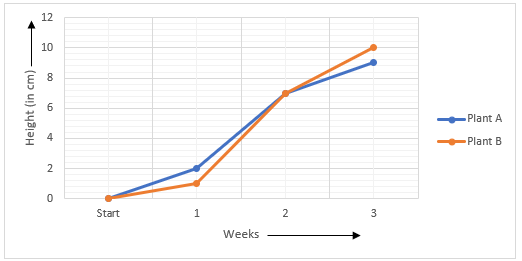a) How high was Plant A after

i) 2 weeks
Sol.: – The plant A was 7 cm high after 2 weeks

ii) 3 weeks
Sol.: – The plant A was 9 cm high after 3 weeks

b) How high was Plant B after

i) 2 weeks
Sol.: – The plant B was 7 cm high after 2 weeks

ii) 3 weeks
Sol.: – The plant B was 10 cm high after 3 weeks

c) How much did Plant A grow during the 3rd week?

Sol.: – Growth of Plant A during 3rd week = Total height in 3rd week – Total height in 2nd week
= 9cm – 7cm
= 2 cm

d) How much did Plant B grow from the end of the 2nd week to the end of the 3rd week?

Sol.: – Growth of Plant B from the end of the 2nd week to the end of the 3rd week = Total height in 3rd week – Total height in 2nd week
= 10cm – 7cm
= 3 cm

e) During which week did Plant A grow most?

Sol.:

Growth of plant A during 1st week = 2 cm – 0 cm = 2 cm
Growth of plant A during 2nd week = 7 cm – 2 cm = 5 cm
Growth of plant A during 3rd week = 9 cm – 7 cm = 2 cm
So, Plant A grew the highest during 2nd week.

f) During which week did Plant B grow least?

Sol.:

Growth of plant B in 1st week = 1 cm – 0 cm = 1 cm
Growth of plant B in 2nd week = 7 cm – 1 cm = 6 cm
Growth of plant B in 3rd week = 10 cm – 7 cm = 3 cm
So, Plant B grew the least during 1st week.

g) Were the two plants of the same height during any week shown here? Specify.

Sol.: – As we can observe from the graph that, the two plants are of same height at the end of the 2nd week.

4. The following graph shows the temperature forecast and the actual temperature for each day of a week.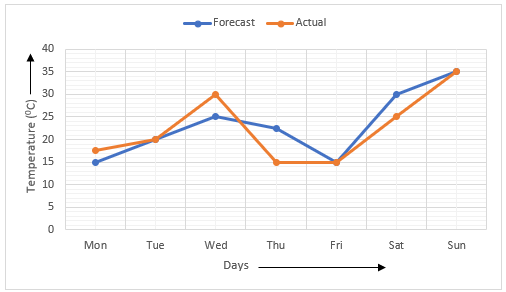a) On which days was the forecast temperature the same as the actual temperature?
Sol.: – On Tuesday, Friday and Sunday the forecast temperature was same as actual temperature.

b) What was the maximum forecast temperature during the week?
Sol.: – The maximum forecast temperature during the week was 35 0C on Sun.

c) What was the minimum actual temperature during the week?
Sol.: – The minimum actual temperature during the week was 15 0C on Thu and Fri.

d) On which day did the actual temperature differ the most from the forecast temperature?
Sol.: – The actual temperature differed the most from the forecast temperature on Thursday.

5) Use the tables below to draw linear graphs.

a) The number of days a hill side city received snow in different years.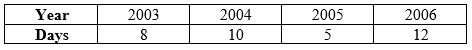Sol.: – By taking, Years on X-Axis and Days on Y-Axis.
2 units of X-Axis = 1 Year
1 unit of Y-Axis = 2 Days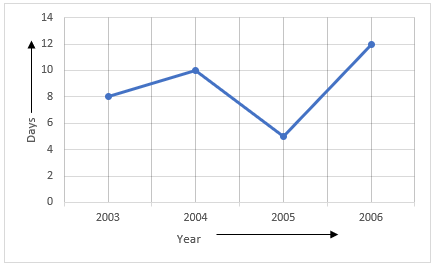b) Population (in thousands) of men and women in a village in different years.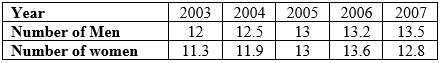Sol.: – By taking, Years on X-Axis and Population (in thousand) on Y-Axis.
2 units of X-Axis = 1 Year
1 unit of Y-Axis = 300 men/women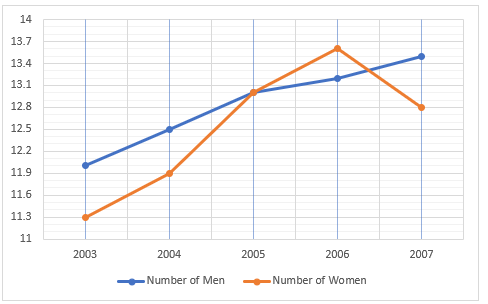6. A courier-person cycles from a town to a neighbouring suburban area to deliver a parcel to a merchant. His distance from the town at different times is shown by the following graph.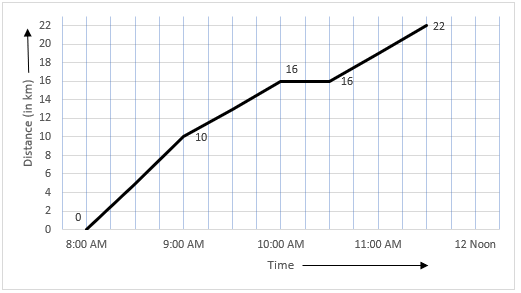a) What is the scale taken for the time axis?
Sol.: – Scale taken for the time axis is 4 unit = 1 Hour.

b) How much time did the person take for the travel?
Sol.: – The person took 3 hours for travel.

c) How far is the place of the merchant from the town?
Sol.: – The merchant is 22 km away from the town.

d) Did the person stop on his way? Explain.
Sol.: – As we can observed from the graph, the distance in not increasing from 10 AM to 10.30 AM. So, the person stopped during that interval.

e) During which period did he ride fastest?
Sol.: – To find during which period he ride fastest, we need to find the interval in which he has travelled the most.
As we observed from the graph, the person has travelled the most during 8 AM to 9 AM.

7. Can there be a time-temperature graph as follows? Justify your answer.

i)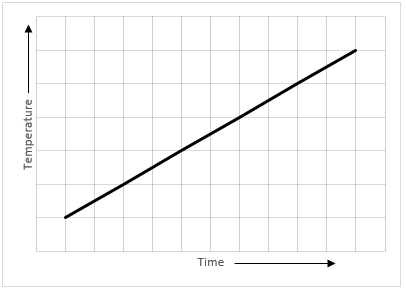Sol.: – This can be a time – temperature graph, it shows that with increase in time temperature also increase.

ii)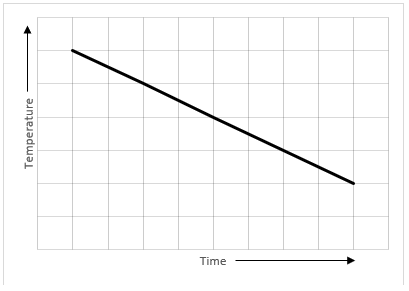Sol.: – This can be a time – temperature graph, it shows that with increase in time temperature decreases.

iii)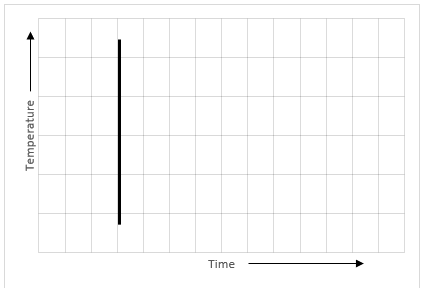Sol.: – This can be not a time – temperature graph, it shows that different temperature is at same time which is not possible.

iv)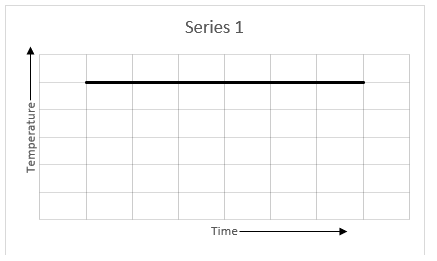Sol.: – This can be a time – temperature graph, it shows that with increase in time temperature remains constant.

The next Exercise for NCERT Solutions for Class 8 Maths Chapter 15 Exercise 15.2 – Introduction to Graphs can be accessed by clicking here

Download NCERT Solutions for Class 8 Maths Chapter 15 Exercise 15.1 – Introduction to Graphs